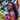# JavaScript Array Methods: forEach vs mapAs a JavaScript developer, you may have been in a position of using forEach or map array method on the `Array.prototype` object to iterate through the elements of an array and wondered the choice to make based on performance gain.

First, let's see MDN's definition of what they do,

forEach() method executes a provided function once for each array element.

map() method creates a new array populated with the results of calling a provided function on every element in the calling array.

To elaborate,

`forEach()` iterates through the elements of an array and perform an operation on each element. It mutates the original data and returns undefined.

`map()` is a functional programming technique. It iterates through the elements of an array, transforms each member of that array, and returns a new array of the same size with the transformed members e.g. performing calculations on a list of numbers. It does not mutate the original data, it returns a new array.

The basic difference between forEach and map is the:

• return value
• data mutation
• chainability
• performance

Given the array;

``const array = [2, 4, 6, 8];``

### Return Value and Data mutation

forEach mutates the original data and has no return value.

``````const newArray = array.forEach((num, index) => {
return array[index] = num * 2;
});

// Expected output: array = [8, 16, 24, 32]
// newArray = undefined``````

Map do not mutate the original data. It returns a new array.

``````const newArray = array.map(num => num * 2);
// Expected output: array = [2, 4, 6, 8]
// newArray = [8, 16, 24, 32]``````

## Chainability

Chainability is a technique used for invoking multiple method calls. forEach is not chainable whereas map is chainable.

Given the array;

``const array = [2, 4, 6, 8];``
``````const newArray = array
.map(num => num * 2)
.filter(num => num > 8)

// Expected output: array = [2, 4, 6, 8]
// newArray = [12, 16]``````

## Performance

In terms of speed, I'll use JSperf, a popular website for checking the performance of JavaScript functions and the DevTools console.

To really see the impact, I'll use a large data set.

Given the array,

``````let array = [];
for (let i = 0; i < 1000000; i++) {
array.push(i)
}``````

Using the DevTools console,

``````// forEach
console.time('forEach');
array.forEach((num, index) => {
return array[index] = num * 2;
});
console.timeEnd('forEach');

// map
console.time('map');
array.map(num => {
return num * 2;
});
console.timeEnd('map');``````

Note that on JSperf, and using the DevTools, `forEach` is faster than `map`. However, if `for` is put into consideration, it is the fastest of all.

forEach map
Return value undefined New array
Data mutation Yes No
Chainability No Yes
Performance More performant Less performant

## Conclusion

map and forEach are methods on the Array.prototype object. For proper usage, consider the scenario to determine the method to use.

When working with functional programming, consider using map to avoid mutating data but when you do not care about data mutation, returning a value or chaining, use forEach.

Article Tag  Performance